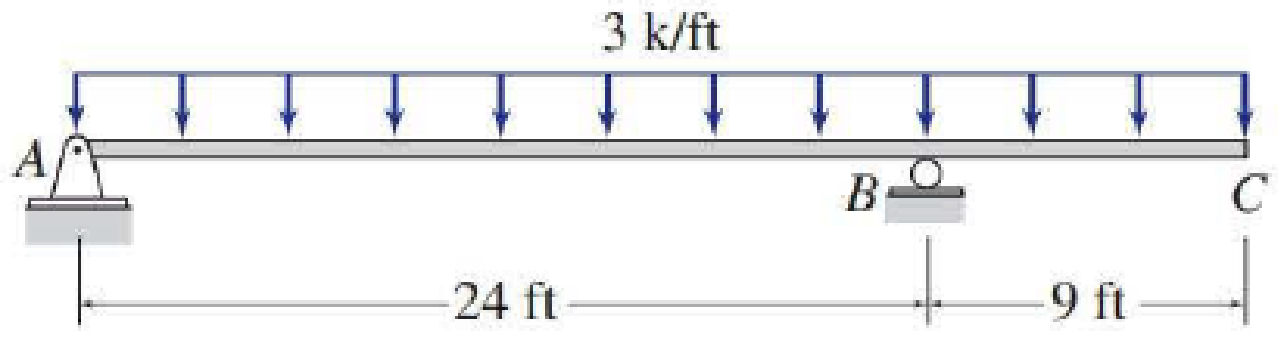# 5.29 through 5.51 Draw the shear and bending moment diagrams and the qualitative deflected shape for the beam shown.

#### Solutions

Chapter
Section
Chapter 5, Problem 38P
Textbook Problem
1 views

## 5.29 through 5.51 Draw the shear and bending moment diagrams and the qualitative deflected shape for the beam shown.To determine

Plot the shear and bending moment diagram and the qualitative deflected shape.

### Explanation of Solution

Sign conversion:

Apply the sign convention for calculating the equations of equilibrium as below.

• For the horizontal forces equilibrium condition, take the force acting towards right side as positive (+) and the force acting towards left side as negative ().
• For the vertical forces equilibrium condition, take the upward force as positive (+) and downward force as negative ().
• For moment equilibrium condition, take the clockwise moment as negative and counter clockwise moment as positive.

Apply the following sign convention for calculating the shear and bending moments.

• When the portion of the beam considered is left of the section, then the external force acting to the left are considered as positive.
• When the portion of the beam considered is right of the section, then the external force acting to the right are considered as positive.
• When the portion of the beam considered is left of the section, then the external force acting upward are considered as positive.
• When the portion of the beam considered is right of the section, then the external force acting downward are considered as positive.
• When the portion of the beam considered is left of the section, then the clockwise moments are considered as positive.
• When the portion of the beam considered is right of the section, then the counterclockwise moments are considered as positive.

Calculation:

Show the free-body diagram of the entire beam as in Figure 1.

Find the horizontal reaction at point A by resolving the horizontal equilibrium.

+Fx=0Ax=0Ax=0

Find the vertical reaction at point B by taking moment about point A.

+MA=03(33)(332)+By(24)=01633.5+24By=0By=68.06k

Find the vertical reaction at point A by resolving the vertical equilibrium.

+Fy=0Ay3(33)+By=0Ay99+68.06=0Ay=30.94k

Show the reactions of the beam as in Figure 2.

Shear diagram:

Point A:

SA,L=0SA,R=30

### Still sussing out bartleby?

Check out a sample textbook solution.

See a sample solution

#### The Solution to Your Study Problems

Bartleby provides explanations to thousands of textbook problems written by our experts, many with advanced degrees!

Get Started

Find more solutions based on key concepts
Same as Problem 4.6-7, but use ASD.

Steel Design (Activate Learning with these NEW titles from Engineering!)

In a commercial water heater, 20 gallons/min of water is heated from 60 F to 140 F. Calculate the amount of ene...

Engineering Fundamentals: An Introduction to Engineering (MindTap Course List)

Define an actor, and provide three examples.

Systems Analysis and Design (Shelly Cashman Series) (MindTap Course List)

What does a user interface control?

Enhanced Discovering Computers 2017 (Shelly Cashman Series) (MindTap Course List)

Wheel A: 32A60H8V Wheel B: 39C100L6V Which wheel is harder?

Precision Machining Technology (MindTap Course List)

List at least five things that should be checked while inspecting a vehicles battery.

Automotive Technology: A Systems Approach (MindTap Course List)

What are the three goals of green computing?

Fundamentals of Information Systems

What else should be on Charlies list?

Principles of Information Security (MindTap Course List)

Explain the need for the two-phase commit protocol. Then describe the two phases.

Database Systems: Design, Implementation, & Management

If your motherboard supports ECC DDR3 memory, can you substitute non-ECC DDR3 memory?

A+ Guide to Hardware (Standalone Book) (MindTap Course List)

List some applications that use medium-pressure piping.

Welding: Principles and Applications (MindTap Course List)